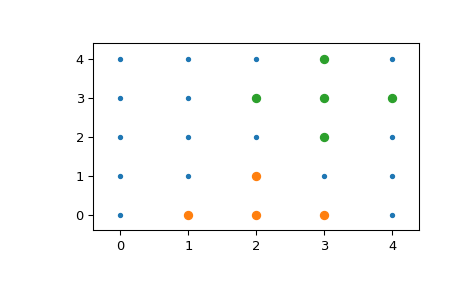# scipy.spatial.KDTree.query_ball_point¶

KDTree.query_ball_point(x, r, p=2.0, eps=0, workers=1, return_sorted=None, return_length=False)[source]

Find all points within distance r of point(s) x.

Parameters
xarray_like, shape tuple + (self.m,)

The point or points to search for neighbors of.

rarray_like, float

The radius of points to return, must broadcast to the length of x.

pfloat, optional

Which Minkowski p-norm to use. Should be in the range [1, inf]. A finite large p may cause a ValueError if overflow can occur.

epsnonnegative float, optional

Approximate search. Branches of the tree are not explored if their nearest points are further than `r / (1 + eps)`, and branches are added in bulk if their furthest points are nearer than `r * (1 + eps)`.

workersint, optional

Number of jobs to schedule for parallel processing. If -1 is given all processors are used. Default: 1.

New in version 1.6.0.

return_sortedbool, optional

Sorts returned indicies if True and does not sort them if False. If None, does not sort single point queries, but does sort multi-point queries which was the behavior before this option was added.

New in version 1.6.0.

return_length: bool, optional

Return the number of points inside the radius instead of a list of the indices.

New in version 1.6.0.

Returns
resultslist or array of lists

If x is a single point, returns a list of the indices of the neighbors of x. If x is an array of points, returns an object array of shape tuple containing lists of neighbors.

Notes

If you have many points whose neighbors you want to find, you may save substantial amounts of time by putting them in a KDTree and using query_ball_tree.

Examples

```>>> from scipy import spatial
>>> x, y = np.mgrid[0:5, 0:5]
>>> points = np.c_[x.ravel(), y.ravel()]
>>> tree = spatial.KDTree(points)
>>> sorted(tree.query_ball_point([2, 0], 1))
[5, 10, 11, 15]
```

Query multiple points and plot the results:

```>>> import matplotlib.pyplot as plt
>>> points = np.asarray(points)
>>> plt.plot(points[:,0], points[:,1], '.')
>>> for results in tree.query_ball_point(([2, 0], [3, 3]), 1):
...     nearby_points = points[results]
...     plt.plot(nearby_points[:,0], nearby_points[:,1], 'o')
>>> plt.margins(0.1, 0.1)
>>> plt.show()
```